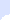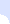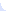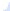# Reseach Projects- Optimal Perturbation Control of General Topology Molecular NetworksHome Research Projects Publications Teaching Students# Optimal Perturbation Control of General Topology Molecular Networks

Abstract:
We develop a comprehensive framework for optimal perturbation control of dynamic networks. The aim of the perturbation is to drive the network away from an undesirable steady-state distribution and to force it to converge towards a desired steady-state distribution. The proposed framework does not make any assumptions about the topology of the initial network, and is thus applicable to general-topology networks. We define the optimal perturbation control as the minimum-energy perturbation measured in terms of the Frobenius-norm between the initial and perturbed probability transition matrices of the dynamic network. We subsequently demonstrate that there exists at most one optimal perturbation that forces the network into the desirable steady-state distribution. In the event where the optimal perturbation does not exist, we construct a family of suboptimal perturbations, and show that the suboptimal perturbation can be used to approximate the optimal limiting distribution arbitrarily closely. Moreover, we investigate the robustness of the optimal perturbation control to errors in the probability transition matrix, and demonstrate that the proposed optimal perturbation control is robust to data and inference errors in the probability transition matrix of the initial network. Finally, we apply the proposed optimal perturbation control method to the Human melanoma gene regulatory network in order to force the network from an initial steady-state distribution associated with melanoma and into a desirable steady-state distribution corresponding to a benign cell.

MATLAB & MATHEMATICA Code:

P_matrix.xls

CODE: The MATLAB code uses CVX, a package for specifying and solving convex programs. One needs to download and install the CVX package before running the MATLAB programs

main.m with output the minimal-perturbation energy matrix.xls

optimal_control.m

The main code is also displayed below:
```0001 %%%%%%%%%%%%%%%%%%%%%%%%%%%%%%%%%%%%%%%%%%%%%%%%%%%%%%%%%%%%%%%%%%%%%%%%%%%%%%%%%%%%%%%%%%%%%%%%%%%%%%%%
0002 %% The  program "main.m" reads a square matrix,
0003 %% the probability transition matrix of the network,
0004 %% "P_matrix.xls" in excel format and returns as output
0005 %% the optimal perturbation matrix "C", which forces
0006 %% the network to transition from its initial steady-state to the desired steady-state pid.
0007 %% This code uses the CVX software. For installation instructions, please
0009
0010 %% Define the variables
0011 %%%%%%%%%%%%%%%%%%%%%%%%
0012
0014 N = size(P0,1);
0015 OneVec = ones(N,1);
0016
0017
0018 %% Define the desired steady-state distribution
0019 %%%%%%%%%%%%%%%%%%%%%%%%%%%%%%%%%%%%%%%%%%%%%%%
0020
0021 pid1 = 0.015525 * ones(64,1);
0022 pid2 = 10^(-4) * ones(64,1);
0023 pid = [pid1;pid2];
0024
0025
0026 %% Call the optimal_control function
0027 %%%%%%%%%%%%%%%%%%%%%%%%%%%%%%%%%%%%%
0028
0029 C = optimal_control(P0,pid);
0030
0031
0032 %% Writing and plotting
0033 %%%%%%%%%%%%%%%%%%%%%%%%
0034
0035 success = xlswrite('Perturbation-Matrix', C);
0036```

P0.xls
P1.xls
P2.xls
Pepsilon.xls
Pa.xls

G0.xls
G1.xls
G2.xls
Gepsilon.xls
Ga.xls